# 调味酱产品

•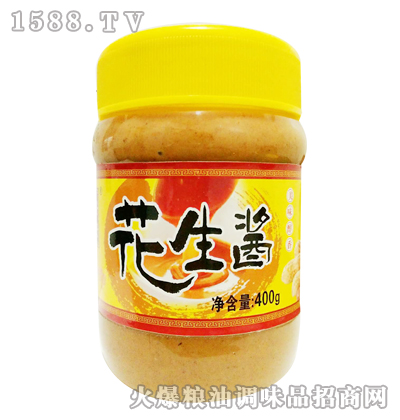我要代理
•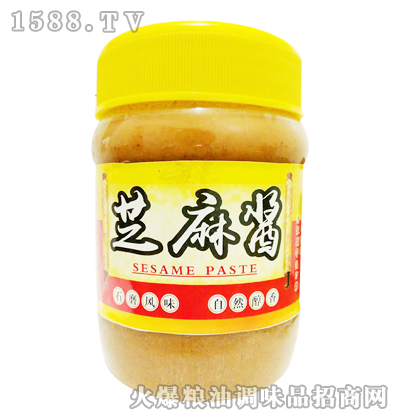我要代理
•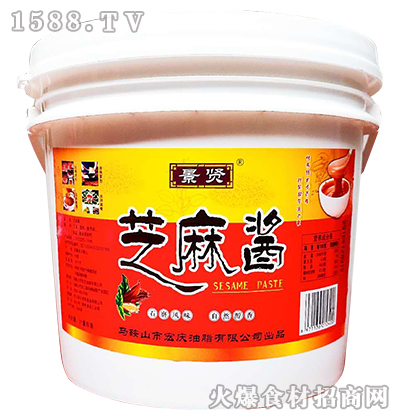我要代理
•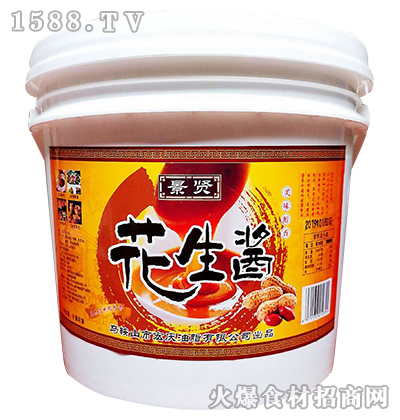我要代理
•我要代理
•我要代理
•我要代理
•我要代理
•我要代理
•我要代理
•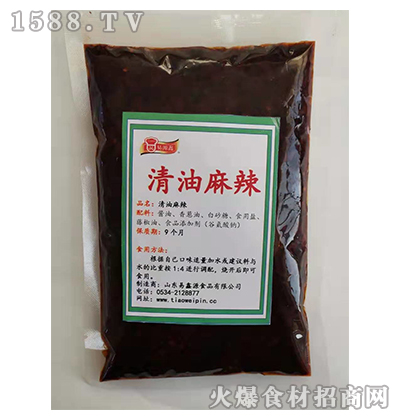我要代理
•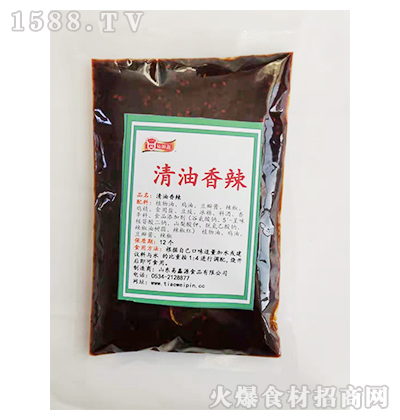我要代理
•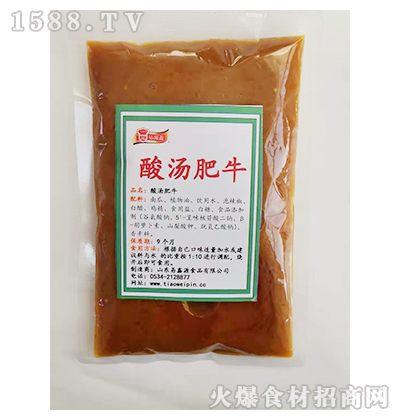我要代理
•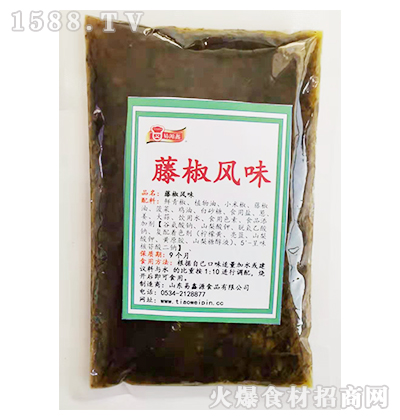我要代理
•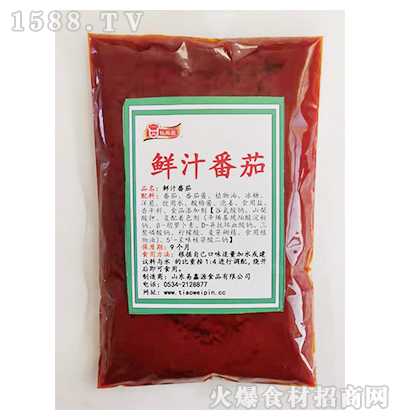我要代理
•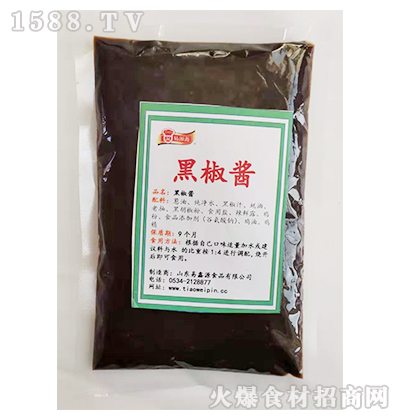我要代理
•我要代理
•我要代理
•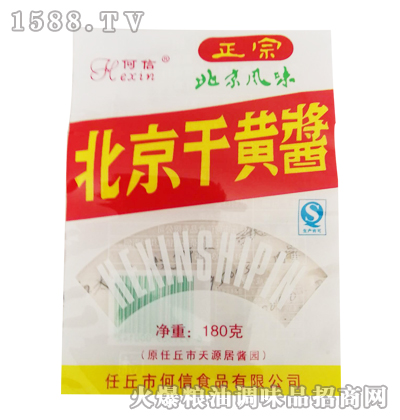我要代理
•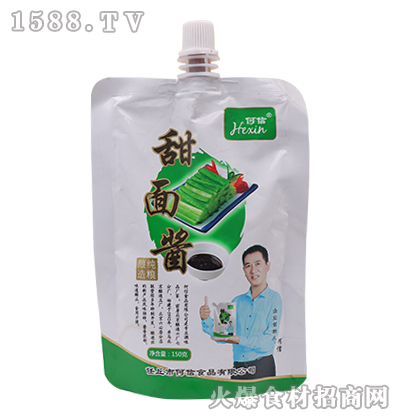我要代理
•我要代理
•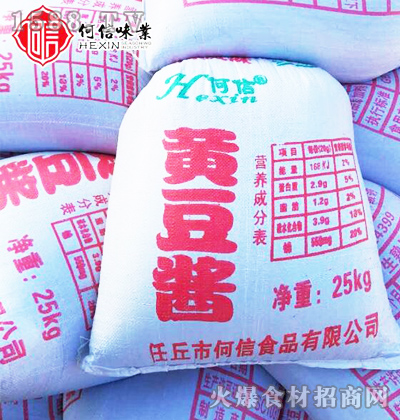我要代理
•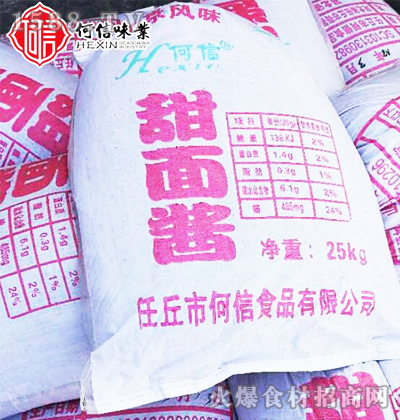我要代理
•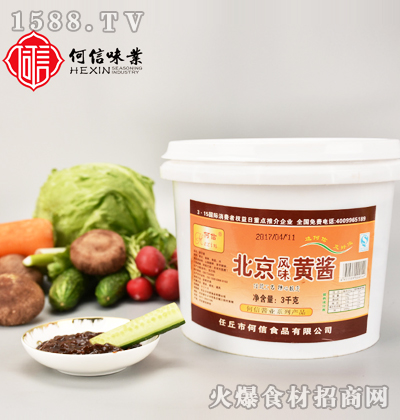我要代理
•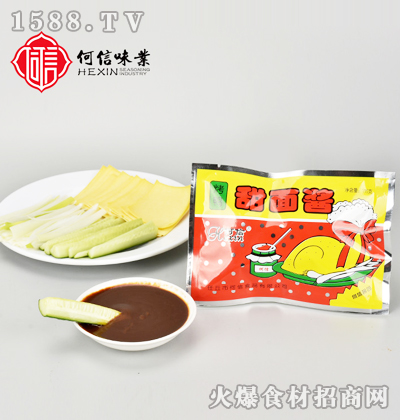我要代理
•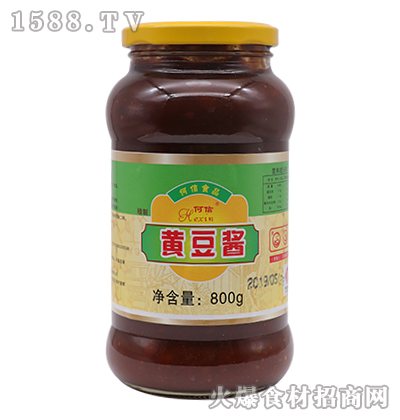我要代理
•我要代理
•我要代理
•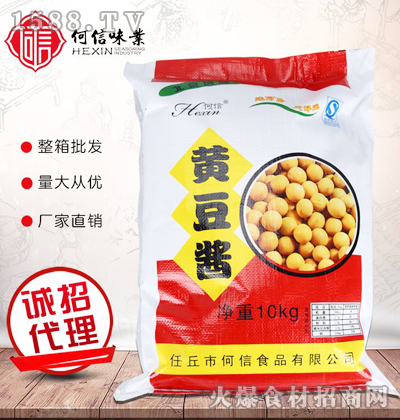我要代理
•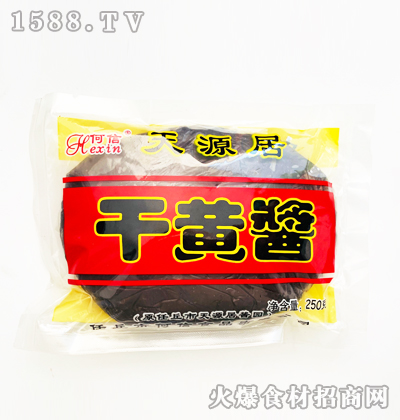我要代理
•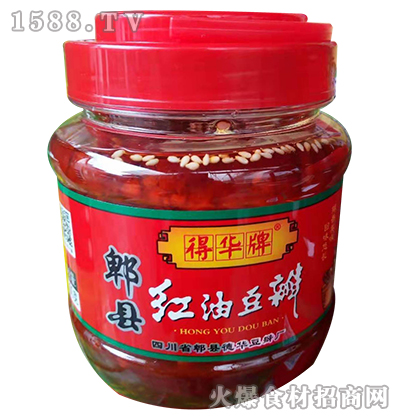我要代理
•我要代理
•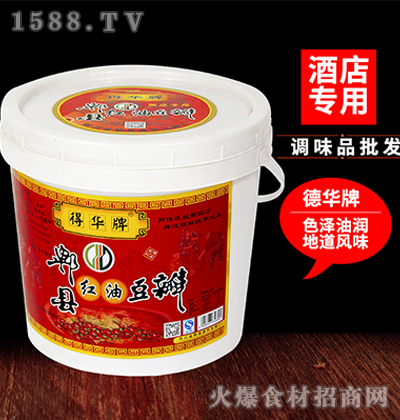我要代理
•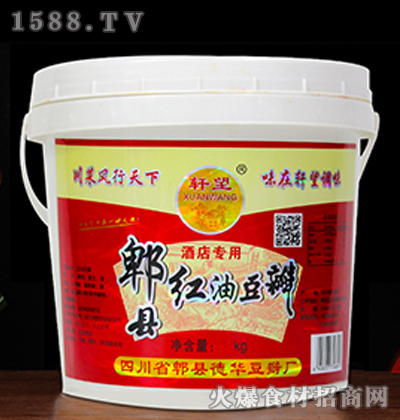我要代理
•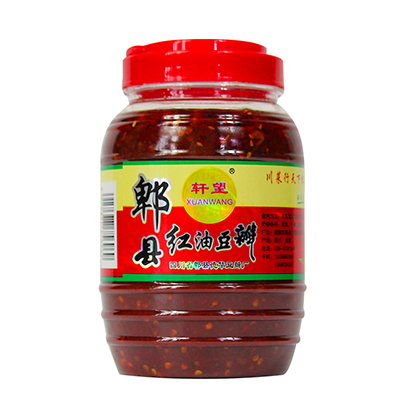我要代理
•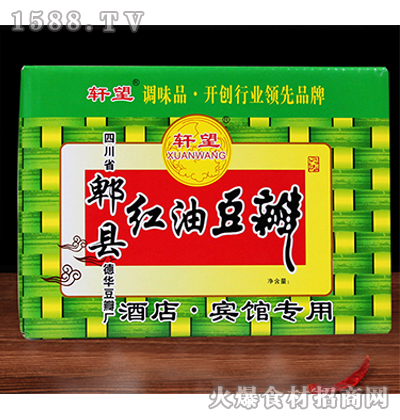我要代理
•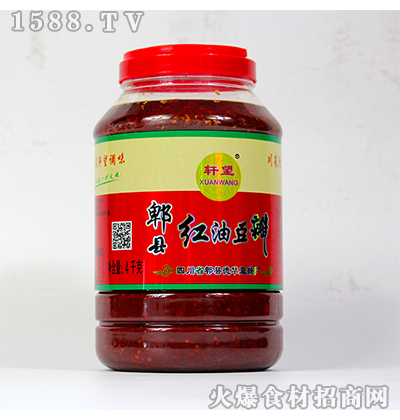我要代理
•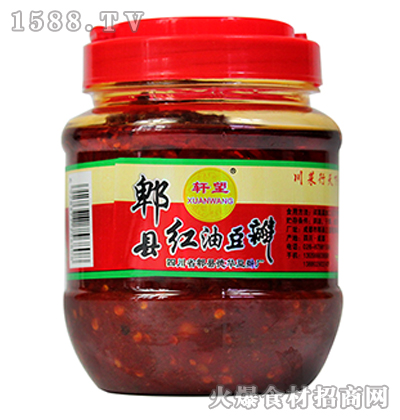我要代理
•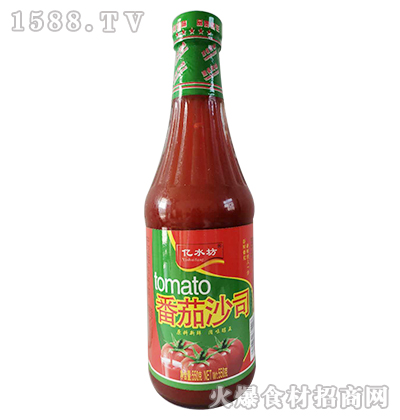我要代理
•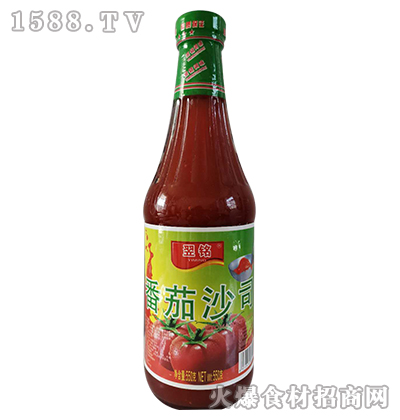我要代理
•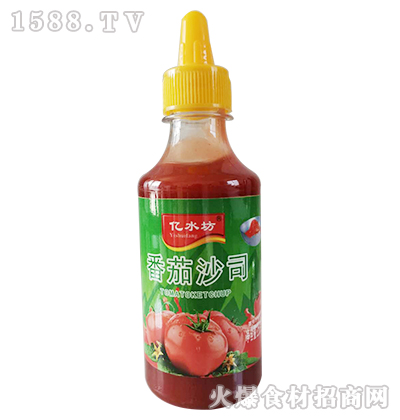我要代理
•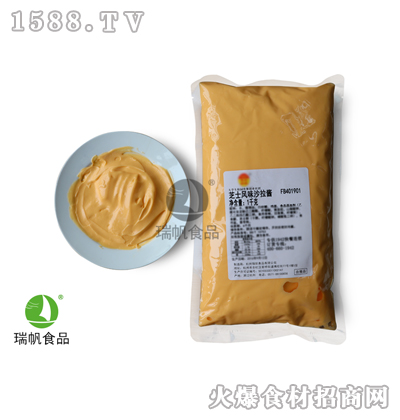我要代理
•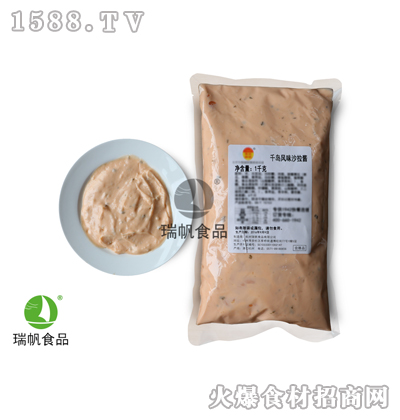我要代理
•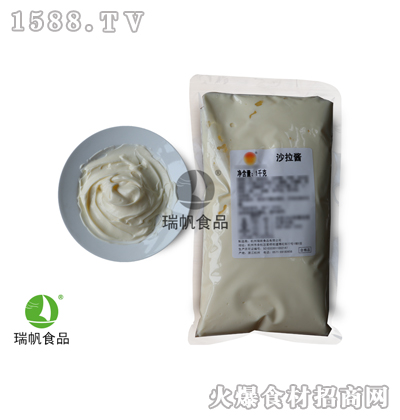我要代理
•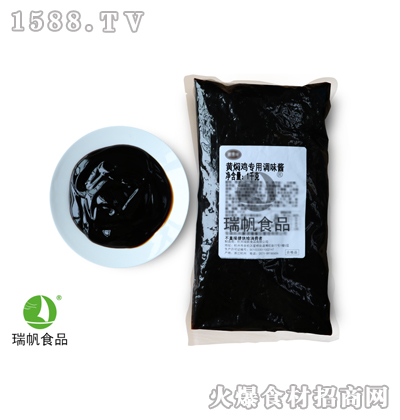我要代理
•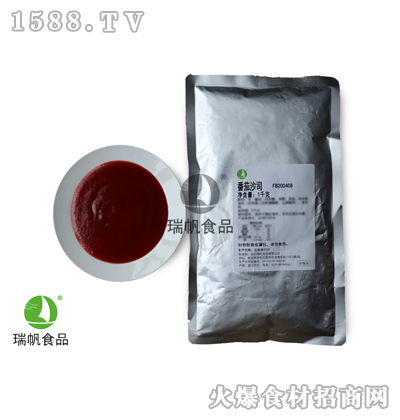我要代理
•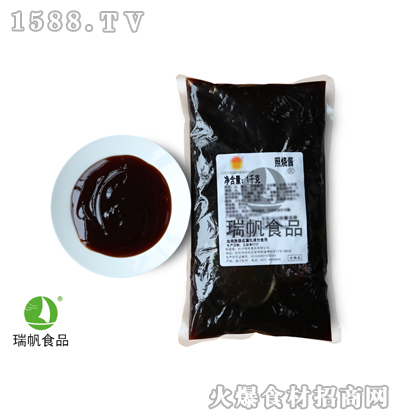我要代理
•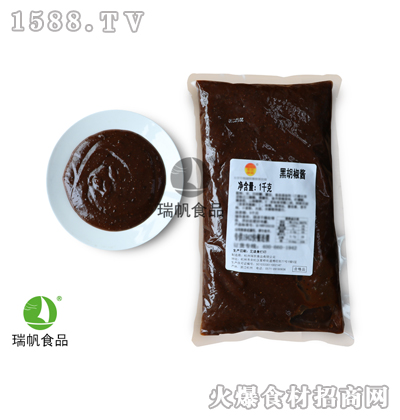我要代理
•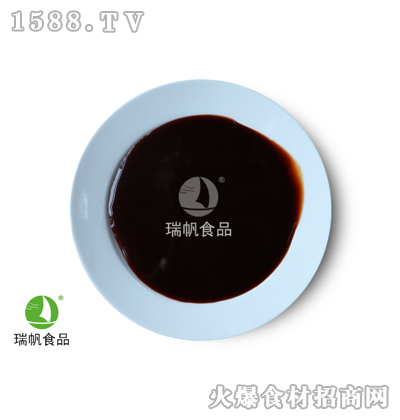我要代理
•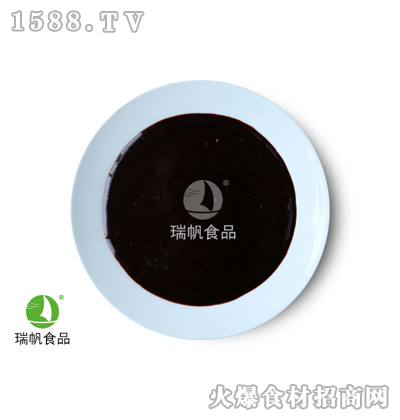我要代理
•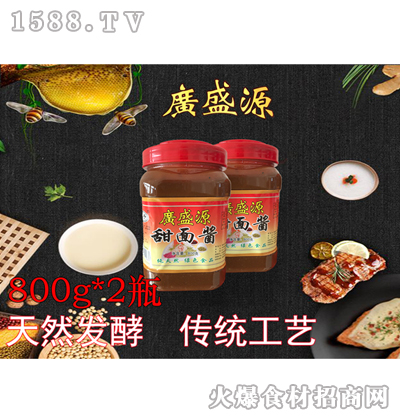我要代理
•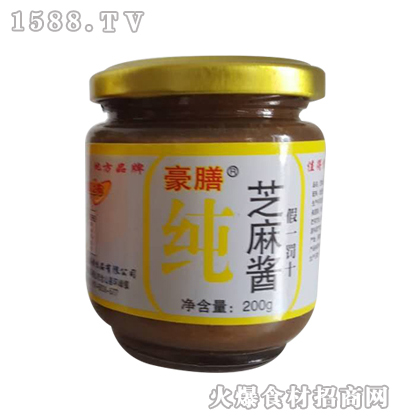我要代理
•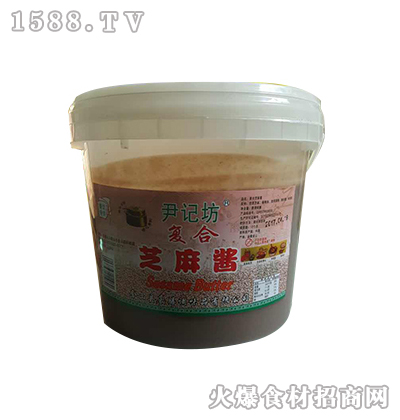我要代理
•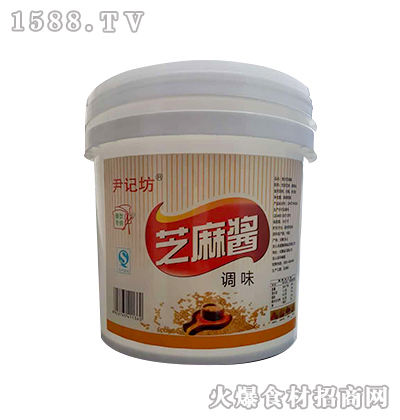我要代理
•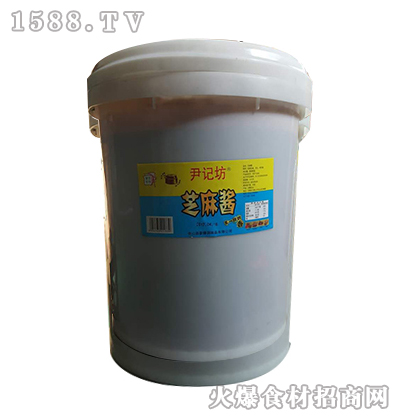我要代理
•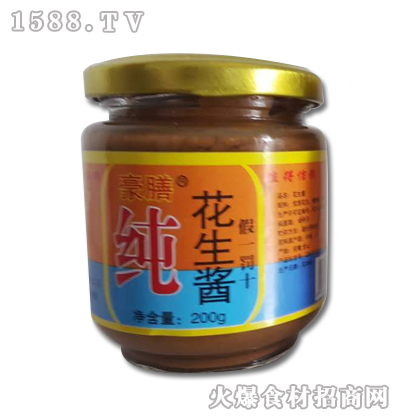我要代理
•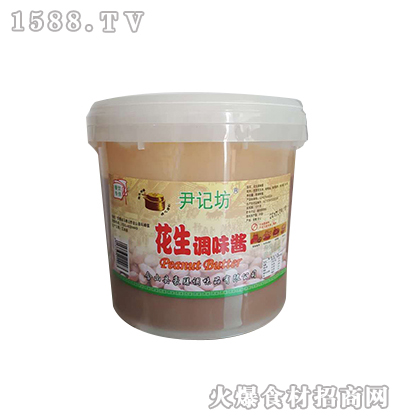我要代理
•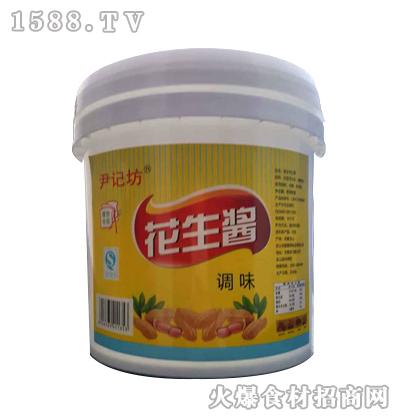我要代理
•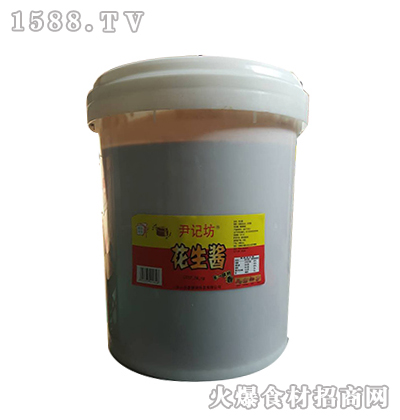我要代理
•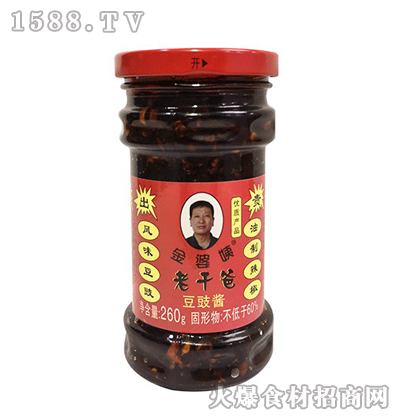我要代理
•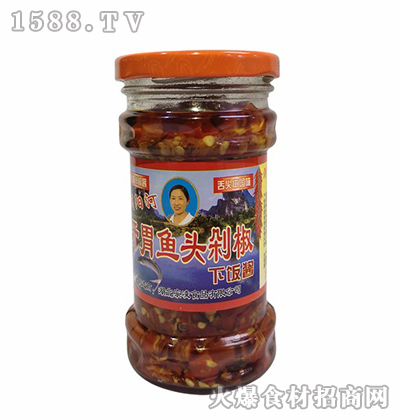我要代理
•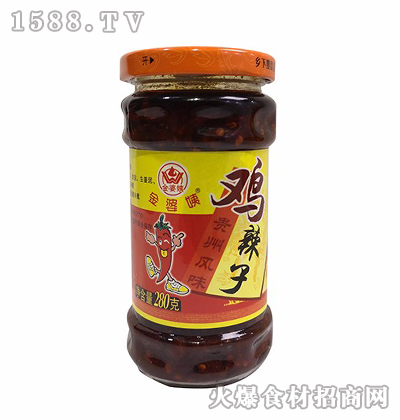我要代理
•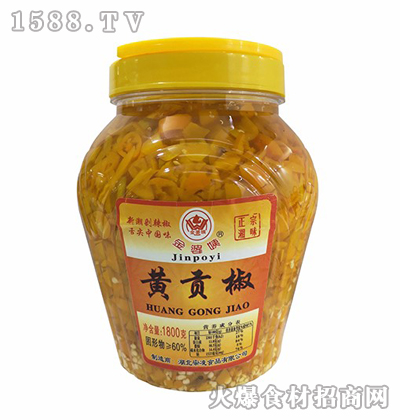我要代理
•我要代理
•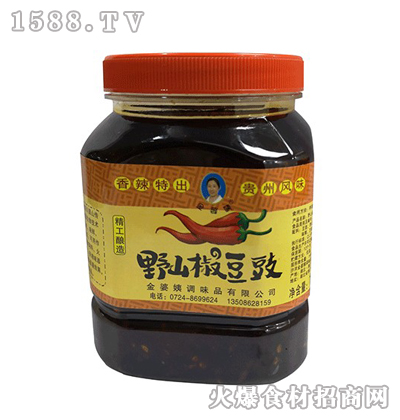我要代理
•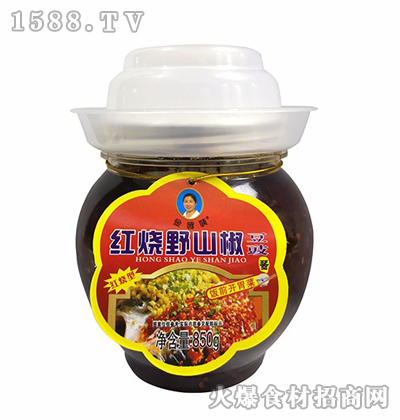我要代理
•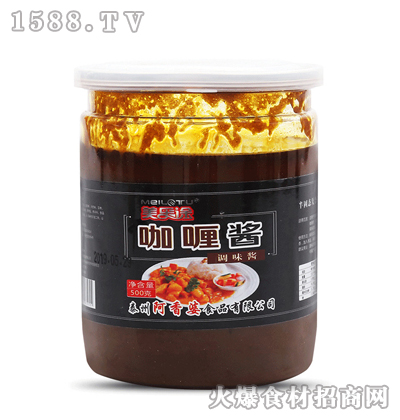我要代理
•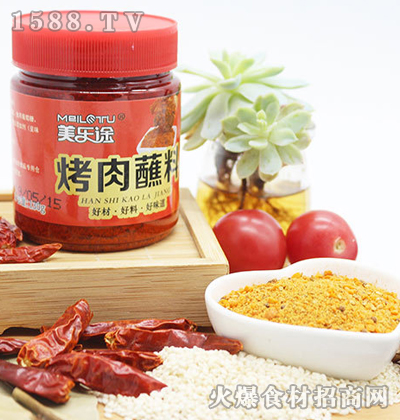我要代理
•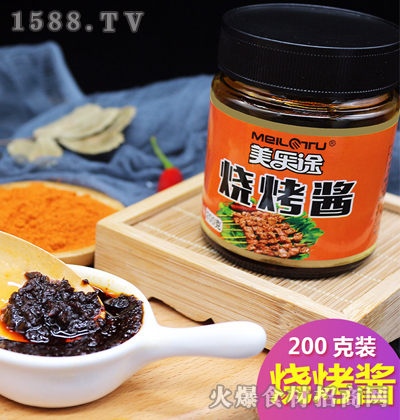我要代理
•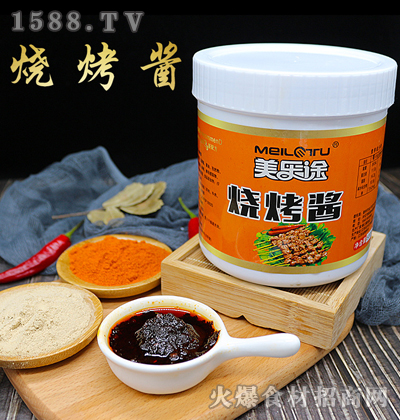我要代理